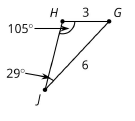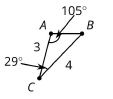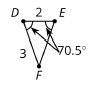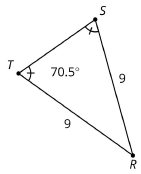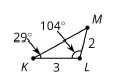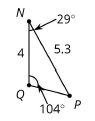# G.3.12.2 Part 2 Corresponding Parts

For each pair of triangles below do the following: 1. write a similarity statement (use ~ and be sure the order is correct) 2. find the scale factor between the similar figures (k = ___ ) 3. give the missing side lengths (ex: AB = ___ or TS = ___ , etc.)